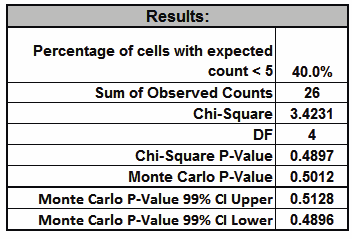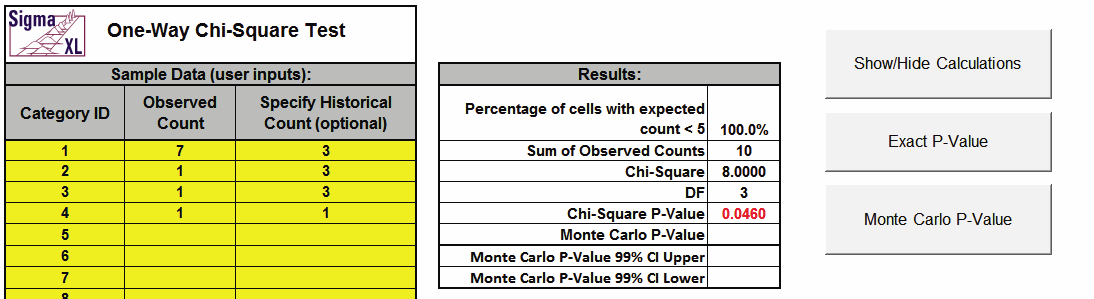Include Top

## Basic Statistical Templates – One-Way Chi-Square Goodness-of-Fit Test - Exact Example

Click SigmaXL > Templates & Calculators > Basic Statistical Templates > One-Way Chi-Square Goodness-of-Fit Test - Exact to access the One Way Chi-Square Goodness-of-Fit Test – Exact calculator. The template gives the following default example.Notes

1. Enter Category ID, Observed Count values and (optional) Historical Counts. Do not modify any other part of this worksheet.
2. If optional historical counts are not specified, chi-square is calculated using equal expected proportions.
3. If optional Historical Counts are specified, a value must be entered for each observed count.
4. The Chi-Square statistic requires that no more than 20% of cells have an expected count less than 5 (and none of the cells have an expected count less than 1). If this assumption is not satisfied, the Chi-Square approximation may be invalid and Exact or Monte Carlo P-Values should be used. In this example 40% of the cells have an expected count less than 5 so Exact should be used.
5. This example shows that the Exact P-Value is 0.5013 and the “large sample” Chi-Square P-Value is 0.4897.
6. Chi-Square Exact solves the permutation problem using enhanced enumeration. For further details refer to the Appendix Exact and Monte Carlo P-Values for Nonparametric and Contingency Test.
7. It is important to note that while exact P-Values are “correct,” they do not increase (or decrease) the power of a small sample test, so they are not a solution to the problem of failure to reject the null due to inadequate sample size.
8. For data that requires more computation time than specified, Monte Carlo P-Values provide an approximate (but unbiased) P-Value that typically matches exact to two decimal places using 10,000 replications. One million replications give a P-Value that is typically accurate to three decimal places. A confidence interval (99% default) is given for the Monte Carlo P-Values.

Monte-Carlo Example

1. The Exact P-Value for this example is solved very quickly, so Monte Carlo is not needed, but we will run it for continuity in the example. Click Monte Carlo P-Value. Select Number of Replications = 10000 and Confidence Level for P-Value = 99%.Tip: The Monte Carlo 99% confidence interval for P-Value is not the same as a confidence interval on the test statistic due to data sampling error. The confidence level for the hypothesis test statistic is still 95%, so all reported P-Values less than .05 will be highlighted in red to indicate significance. The 99% Monte Carlo P-Value confidence interval is due to the uncertainty in Monte Carlo sampling, and it becomes smaller as the number of replications increases (irrespective of the data sample size). The Exact P-Value will lie within the stated Monte Carlo confidence interval 99% of the time.

1. Click OK. ResultsThe Monte Carlo P-Value here is 0.5012 with a 99% confidence interval of 0.4896 to 0.5128. This will be slightly different every time it is run (the Monte Carlo seed value is derived from the system clock). The true Exact P-Value = 0.5013 lies within this confidence interval.

Small Sample Exact Sample

1. Now we will consider a small sample problem. Enter the following values for sample data in the yellow highlight region. Note that the displayed Monte Carlo (or Exact) P-Values are cleared when new data is entered in the template:This example is adapted from Mehta, C.R. and Patel, N. R., IBM SPSS Exact Tests, IBM Corp., page 44.

1. Click Exact P-Value. Select Time Limit for Exact Computation = 60 seconds.2. Click OK. Results:Note that the Exact P-Value is 0.0523 which is a “fail-to-reject” of the null hypothesis (H0), but the “large sample” or “asymptotic” Chi-Square P-Value incorrectly rejected H0 with a P-Value of 0.046. The exact P-Value matches that given in the reference. The error can also go the other way, where a large sample Chi-Square P-Value is a “fail-to-reject” of the null hypothesis and the Exact P-Value is a rejection of H0. In conclusion, always use the Exact (or Monte Carlo) P-Value when the Chi-Square large sample assumptions are not met.

# Web Demos

Our CTO and Co-Founder, John Noguera, regularly hosts free Web Demos featuring SigmaXL and DiscoverSim